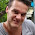## 2/12/2013

### Parallel evaluation

The limited shared memory and thread divergence issues make it difficult the achieve efficient parallellism at the thread level. Therefore, a viable alternative is to let all the threads in a warp work on one position. Then there is more memory per position and the divergence is not an issue, because all the threads in a warp follow the same execution path. The challenge is to find enough to do for all the threads. So, how to take care of this? In the following I present my initial thoughts.

Most of the nodes are leaf nodes. Imagine a search tree where the branching factor is constant 2. Then it is easy to see that the number of nodes per level follows the series: 1, 2, 4, 8, 16, 32, 64, 128, .... The sum of all the previous numbers is the last number minus one. It follows from this observation that most of the nodes in this tree are leaf nodes. With a larger branching factor the ratio of leaf nodes to other nodes is even larger. Although there are cases where the local branching factor is one, it is a safe assumption that a typical chess search tree consists mostly of leaf nodes. We can utilize this piece of information by doing the evaluation in parallel. Evaluation function is mostly called at leaf nodes, so doing it faster helps the overall performance.

If we have an array of pieces stored, we could just let each thread handle one element in the array, i.e. one piece. In the end game, most of the threads do nothing then. That does not sound too good. In addition, the evaluation methods for different pieces differ from each other. Using threads for the elementary bit operations on the bitboards is not wise either, because the GPU has native lsb and popcount-instructions, for finding the least significant bit and calculating the number of bits, respectively. I am not even sure if I will eventually use bitboards. It is probably better if the threads work together on the different features of the position that are somewhat larger.

I have come up with an evaluation function that, in its simplicity, could look something like:

```int Board::evaluate ()
{
unsigned int score = 0;
evaluate_targets ();
for (int i = 0; i < number_of_pieces; i++)
{
int j = pieces[i].getPieceType ();
int k = pieces[i].getPosition ();
score += material_score[j];
score += placement_score[j][k];
}
return score;
}
```

This seems pretty simple. Every piece contributes to the total score its material value and its placement score. The trick is that the placement score table is dynamic. It depends mostly on the pawn structure and the position of the king, and it is calculated in evaluate_targets()-function. Its values could be stored in a hash table, but more probably it is cheaper to calculate them in parallel, because the hash table will not fit into shared memory.

I have had no time to think this more thoroughly yet, but I will do that in the near future.

#### 2 comments:

1.Nice, I'm curious about the development. Doing the evaluation in parallel does require breadth first search. Will you be able to hold that in memory?

2.I noticed that I have explained the idea poorly. I did not mean breadth first search. I am using a variation of the alpha-beta-pruning (i.e. depth first search), but I let all the threads in a warp go in one branch. Then, at a leaf node, the threads can do separate tasks for the evaluation in parallel. So I handle one leaf node at a time, but with multiple threads doing the calculation in it.

I am currently testing completely different things, but I'll write about them later.